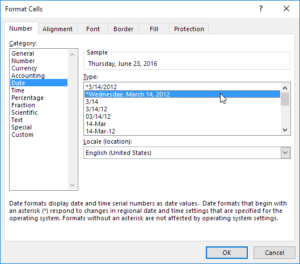# 3 secrets about Excel time management

Excel time management often causes a despair: instead of a date, a number is displayed, e.g. 41615. It depends on the cellformat.

Excel stores dates as a serial number. By default, January 1, 1900 is equivalent to number 1, and for example, 1st January 2013 is 41275, or 22th September 2017 is 43000, and 18th July 2020 will be 44000!

## Change a cell’s number format to DateFirst select the cell(s) containing the numbers.
Then press Ctrl 1 to open Format Cells dialog box.

In the Number group choose ‘Date’ and then select the desired format.

Check the Spam box above, to see if it fits to your idea.

The fact that dates are numbers adds up to 3 more exciting questions:

## 1. What is the first and last date you can enter in Excel?

The first number is zero, which is 00-01-1900, and the first date (1) belongs to 1st January, 1900.

The last date is 31-12-9999, which is numbered 2958465!

With this nearly 3 million date, you can make simply calculations, and know further information about each day, such as which day of the week it is.

Of course, you can record any previous or later date as a text, but you can not count with them.

## 2. Excel time management: what about decimal places?

Since coding is 1 = 1 day = 24 hours, then 0.5 = 12 hours, 0.75 = 18:00 and 1/3 = 0.33333 day = 8 hours.Rounding should be treated cautiously as 0.001 = 1 minute 26 seconds.

One day is (24 x 60 x 60 = ) 86.400 seconds, so one second (1/86400) is 0.0000116.

This is due to the fact that not only the days but also the dates can be counted. For example, you can calculate your work time record by subtracting departure and arrival time, e.g.: 17: 00 – 8: 00 = 9:00

To see time in a correct way, choose from Format Cells (Ctrl 1) / Time options.

Tip: Do you need to record the exact time in a cell? Press Ctrl Shift : buttons, and it will insert current time in the cell.

## 3. How to format the 24:00 or more hours?

If you choose Time format, you won’t see days. This can be a problem, if you have more than 24 hours, for example, when working hours are aggregated.

To see the exact hours, use Custom Format in the Cell Formatting window, and change h:mm to [h]:mm

## Do you have other questions about Excel dates?

For more information on Excel time and date formats, visit Microsoft’s page. If you want to get specific exercises and get personalized tips, take a private Excel course!